### Home > AC > Chapter 10 > Lesson 10.4.2 > Problem10-139

10-139.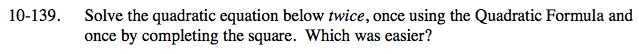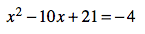$x=\frac{-b\pm\sqrt{b^2-4ac}}{2a}$

$x=\frac{-10\pm\sqrt{10^2-4(1)(25)}}{2(1)}$

Solve for x.

$x=\frac{-10\pm\sqrt{100-100}}{2}$

$x=\frac{-10}{2}$

x = −5

Complete the square.

Subtract 21 from both sides.

x2 − 10x = −25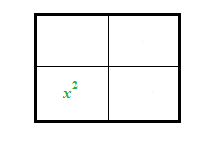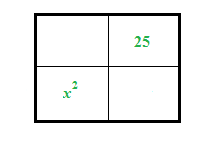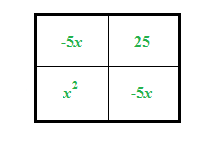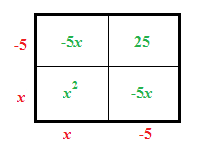Draw a generic rectangle.

You need 25 to complete the square.

Complete the rectangle.

Factor.

(x − 5)2 = −25 + 25

(x − 5)2 = 0

Solve for x.

$\sqrt{(x-5)^2}=\sqrt{0}$

x − 5 = 0

x = 5